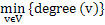Related Articles
GATE | GATE-CS-2003 | Question 40
• Last Updated : 10 Oct, 2014

A graph G = (V, E) satisfies |E| ≤ 3 |V| – 6. The min-degree of G is defined as. Therefore, min-degree of G cannot be
(A) 3
(B) 4
(C) 5
(D) 6

Answer: (D)

Explanation:

Let the min-degree of G be x, then G has at least |v| *x/2 edges.

|v|*x/2 <= 3* |v| -6

for x=6, we get 0 <= -6, Therefore, min degree of G cannot be 6.

Hence answer is (D).My Personal Notes arrow_drop_up